NEET  >  NCERT Exemplar: Equilibrium

# NCERT Exemplar: Equilibrium - Notes | Study Chemistry Class 11 - NEET

 1 Crore+ students have signed up on EduRev. Have you?

MULTIPLE CHOICE QUESTIONS - I

Q.1. We know that the relationship between Kc and Kp is
Kp = Kc (RT)∆n
What would be the value of  ∆n  for the reaction
NH4Cl (s) ⇌ NH3 (g) + HCl (g)
(1) 1
(2) 0.5
(3) 1.5
(4) 2
Ans.
(2)
Solution.

The relationship between Kp and Kc is
Kp = Kc (RT)∆n
Where ∆n = (number of moles of gaseous products) – (number of moles of gaseous reactants)
For the reaction,
NH4Cl (s) ⇌ NH3 (g) + HCl (g)
∆n = 2 – 0 = 2

Q.2. For the reaction H2(g) + I2(g) ⇌ 2HI (g), the standard free energy is ΔGθ > 0. The equilibrium constant (K ) would be __________.
(1) K = 0
(2) K > 1
(3) K = 1
(4) K < 1
Ans.
(4)
Solution.

∆G° = -RTln K. ∆G° > 0 means ∆G° is +ve. This can be so only if In K is -ve, i.e., K< 1.

Q.3. Which of the following is not a general characteristic of equilibria involving physical processes?
(1) Equilibrium is possible only in a closed system at a given temperature.
(2) All measurable properties of the system remain constant.
(3) All the physical processes stop at equilibrium.
(4) The opposing processes occur at the same rate and there is dynamic but stable condition.
Ans.
(3)
Solution.

All the physical processes like melting of ice and freezing of water, etc., do not stop at equilibrium.

Q.4. PCl5, PCl3 and Cl2 are at equilibrium at 500K in a closed container and their concentrations are 0.8 × 10–3 mol L–1, 1.2 × 10–3 mol L–1 and 1.2 × 10–3 mol L–1 respectively. The value of Kc for the reaction PCl5 (g) ⇌ PCl3 (g) + Cl2 (g) will be
(i) 1.8 × 103 mol L-1
(ii) 1.8 × 10-3
(iii) 1.8 × 10-3 L mol-1
(iv) 0.55 × 104
Ans.
(2)
Solution.

PCl5(g)   ⇌ PCl3(g) + Cl2(g)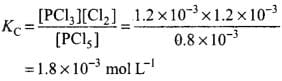Q.5. Which of the following statements is incorrect?
(1) In equilibrium mixture of ice and water kept in perfectly insulated flask mass of ice and water does not change with time.
(2) The intensity of red colour increases when oxalic acid is added to a solution containing iron (III) nitrate and potassium thiocyanate.
(3) On addition of catalyst the equilibrium constant value is not affected.
(4) Equilibrium constant for a reaction with negative ∆H value decreases as the temperature increases.
Ans.
(2)
Solution.
Fe3++SCN ⇌ FeSCN2+(Red)
When oxalic acid is added to a solution containing iron nitrate and potassium thiocyanate, oxalic acid reacts with Fe3+ ions to form a stable complex ion [Fe(C2O4)3]3-, thus, decreasing the concentration of free Fe3+ ions which in mm decreases the intensity of red colour.
Fe3+ + SCN⇌ [Fe(SCN)]2+ (Red)

Q.6. When hydrochloric acid is added to cobalt nitrate solution at room temperature, the following reaction takes place and the reaction mixture becomes blue. On cooling the mixture it becomes pink. On the basis of this information mark the correct answer.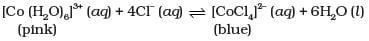(1) ΔH > 0 for the reaction
(2) ΔH < 0 for the reaction
(3) ΔH = 0 for the reaction
(4) The sign of  ΔH cannot be predicted on the basis of this information.

Ans. (1)
Solution.
Since the reaction shifts to backward direction on cooling, this means that the backward reaction is exothermic reaction. Therefore, the forward reaction is endothermic reaction and ∆H > 0.

Q.7. The pH of neutral water at 25°C is 7.0. As the temperature increases, ionisation of water increases, however, the concentration of H+ ions and OH ions are equal. What will be the pH of pure water at 60°C?
(1) Equal to 7.0
(2) Greater than 7.0
(3) Less than 7.0
(4) Equal to zero
Ans.
(3)
Solution.

At 25°C, [H+] = [OH] = 10 7 and Kw = [H+] [OH-] = 10-14. On heating, Kw increases, i.e., [H+] [OH] > 10-14 As [H+] = [OH], [H+]2 > 10-14 or [H+] > 10-7 M or pH < 7.

Q.8. The ionisation constant of an acid, Ka, is the measure of strength of an acid. The Kvalues of acetic acid, hypochlorous acid and formic acid  are 1.74 × 10–5, 3.0 × 10–8 and 1.8 × 10–4 respectively. Which of the following orders of pH of 0.1 mol dm–3 solutions of these acids is correct?
(1) Acetic acid > hypochlorous acid > formic acid
(2) Hypochlorous acid > acetic acid > formic acid
(3) Formic acid > hypochlorous acid > acetic acid
(4) Formic acid > acetic acid > hypochlorous acid
Ans.
(4)
Solution.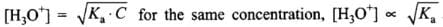But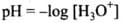Larger the value of Ka, larger will be [H3O+] and lower will be pH.

Q.9.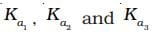are the respective ionisation constants for the following
reactions.
H2S ⇌ H+ + HS-
HS ⇌ H+ + S2-
H2S ⇌ 2H+ + S2-
The correct relationship betweenis
(i)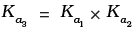(ii)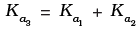(iii)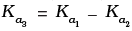(iv)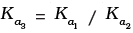Ans.
For the reaction. H2S ⇌ H+ + HS-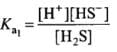For the reaction,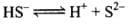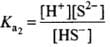When the above two reactions are added, their equilibrium constants are multiplied. Thus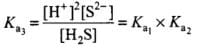Hence, Ka3 = Ka1 x Ka2

Q.10.Acidity of BF3 can be explained on the basis of which of the following concepts?
(1) Arrhenius concept
(2) Bronsted Lowry concept
(3) Lewis concept
(4) Bronsted Lowry as well as Lewis concept.
Ans.
(3)
Solution.

According to Lewis concept, a positively charged or an electron deficient species acts as Lewis acid. BF3 is an electron deficient compound with B having 6 electrons only.

Q.11.Which of the following will produce a buffer solution when mixed in equal volumes?
(1) 0.1 mol dm–3 NH4OH and 0.1 mol dm–3 HCl
(2) 0.05 mol dm–3 NH4OH and 0.1 mol dm–3 HCl
(3) 0.1 mol dm–3 NH4OH and 0.05 mol dm–3 HCl
(4) 0.1 mol dm–3 CH4COONa and 0.1 mol dm–3 NaOH
Ans.
(3)
Solution.

In (3), all HCl will be neutralized and NH4Cl will be formed. Also some NH4OH will be left unneutralized. Thus, the final solution will contain NH4OH and NH4Cl and hence will form a buffer.

Q.12.In which of the following solvents is silver chloride most soluble?
(i) 0.1 mol dm–3 AgNO3 solution
(ii) 0.1 mol dm–3 HCl solution
(iii) H2O
(iv) Aqueous ammonia
Ans.
(4)
Solution.
Silver chloride forms a soluble complex with aqueous ammonia.
AgCl + 2NH3→ [Ag(NH3)2]Cl

Q.13. What will be the value of pH of 0.01 mol dm–3 CH3COOH (Ka = 1.74 × 10–5 )?
(1) 3.4
(2) 3.6
(3) 3.9
(4) 3.0

Ans.(a)
Solution.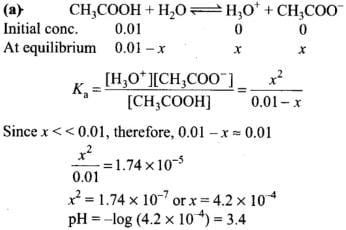Q.14. Ka for CH3COOH is 1.8 × 10–5 and Kb for NH4OH is 1.8 × 10–5 . The pH of ammonium acetate will be
(1) 7.005
(2) 4.75
(3) 7.0
(4) Between 6 and 7
Ans.
(3)
Solution.

Ammonium acetate is a salt of weak acid and weak base.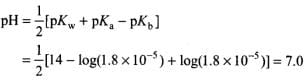Q.15.Which of the following options will be correct for the stage of half completion of the reaction A ⇌ B.
(1) ΔGθ = 0
(2) ΔGθ > 0
(3) ΔGθ< 0
(4) ΔGθ = –RT ln2
Ans.
(1)
Solution.

A ⇌B
ΔG° = -RT In K
At the stage of half completion of reaction [A] = [B],
Therefore, K = 1. Thus, ΔG° = 0.

Q.16.On increasing the pressure, in which direction will the gas phase reaction proceed to re-establish equilibrium, is predicted by applying the Le Chatelier’s principle. Consider the reaction.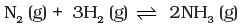Which of the following is correct, if the total pressure at which the equilibrium is established, is increased without changing  the temperature?
(1) K will remain same
(2) K will decrease
(3) K will increase
(4) K will increase initially and decrease when pressure is very high

Ans.(1)
Solution.
N2(g) + 3H2(g) ⇌2NH3(g)
According to Le Chatelier’s principle, at constant temperature, the equilibrium composition will change but K will remain same.

Q.17.What will be the correct order of vapour pressure of water, acetone and ether at 30°C. Given that among these compounds, water has maximum boiling point and ether has minimum boiling point?
(1) Water < ether < acetone
(2) Water < acetone < ether
(3) Ether < acetone < water
(4) Acetone < ether < water
Ans.
(2)
Solution.

Greater the boiling point, less is the vapour pressure. Hence, the correct order of vapour pressures will be:
water < acetone < ether.

Q.18.At 500 K, equilibrium constant, Kc , for the following reaction is 5.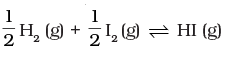What would be the equilibrium constant Kfor the reaction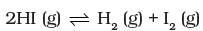(1) 0.04
(2) 0.4
(3) 25
(4) 2.5

Ans.(1)
Solution.
For the reaction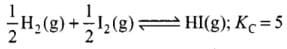For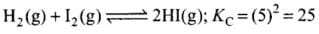For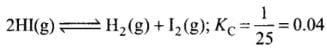Q.19. In which of the following reactions, the equilibrium remains unaffected on addition of small amount of argon at constant volume?
(1) H2 (g) + I2 (g) ⇌ 2HI (g)
(2) PCl5 (g) ⇌ PCl3 (g) + Cl2 (g)
(3) N(g) + 3H2 (g) ⇌ 2NH3 (g)
(4) The equilibrium will remain unaffected in all the three cases.
Ans.
(4)
Solution.

The equilibrium will remain unaffected in all three cases on addition of small amount of inert gas at constant volume.

MULTIPLE CHOICE QUESTIONS - II

In the following questions two or more options may be correct.
Q.20. For the reaction N2O4 (g) ⇌ 2NO2 (g), the value of K is 50 at 400 K and 1700 at 500 K. Which of the following options is correct?
(1) The reaction is endothermic
(2) The reaction is exothermic
(3) If NO2 (g) and N2O4(g) are mixed at 400 K at partial pressures  20 bar and 2 bar respectively, more N2O4 (g) will be formed.
(4) The entropy of the system increases.
Ans.
(1, 3, 4)
Solution.

(1) As the value of K increases with increase of temperature and K = Kf / Kb, this means that kf increases, i.e., forward reaction is favoured. Hence, reaction is endothermic.
(3) At 400 K,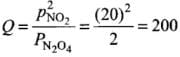Thus, Q > K. Equilibrium will shift backward to form more N2O4.
(4) As reaction is accompanied by increase in the number of moles, entropy increases.

Q.21. At a particular temperature and atmospheric pressure, the solid and liquid phases of a pure substance can exist in equilibrium. Which of the following term defines this temperature?
(1) Normal melting point
(2) Equilibrium temperature
(3) Boiling point
(4) Freezing point
Ans.
(1, 4)
Solution.

These are normal melting point and freezing point since they are measured at atmospheric pressure.

Q.22.The ionisation of hydrochloric in water is given below:
HCl(aq) + H2O (l) ⇌ H3O+ (aq) + Cl- (aq)
Label two conjugate acid-base pairs in this ionisation.

Ans.

 HCl (Acid) Cl– (Conjugate base) H2O (Base) H3O+ (Conjugate acid)

Q.23.The aqueous solution of sugar does not conduct electricity. However, when sodium chloride is added to water, it conducts electricity. How will you explain this statement on the basis of ionisation and how is it affected by concentration of sodium chloride?
Ans.
(i) Sugar being a non-electrolyte does not ionize in water, whereas NaCl ionizes completely in water and produces Na+ and Cl ions which help in the conduction of electricity.
(ii) When concentration of NaCl is increased, more Na+ and Cl ions will be produced. Hence, conductance increases.

Q.24.BF3 does not have proton but still acts as an acid and reacts with NH3. Why is it so? What type of bond is formed between the two?
Ans.
BF3 is an electron deficient compound. Hence, it acts as Lewis acid. NH3 has a lone pair of electrons. Hence, acts as Lewis base. A coordinate bond is formed between the two.
H3N: →BF3

Q.25. Ionisation constant of a weak base MOH, is given by the expression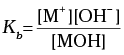Values of ionisation constant of some weak bases at a particular temperature are given below:

 Base Dimethylamine Urea Pyridine Ammonia Kb 5.4 × 10–4 1.3 × 10–14 1.77 × 10–9 1.77 × 10–5

Arrange the bases in decreasing order of the extent of their ionisation at equilibrium. Which of the above base is the strongest?
Ans.Greater is the ionization constant (Kb) of a base, greater is the ionization of the base. Order of extent of ionization at equilibrium is dimethylamine > ammonia > pyridine > urea. Dimethylamine is the strongest base due to maximum value of Kb.

Q.26.Conjugate acid of a weak base is always stronger. What will be the decreasing order of basic strength of the following conjugate bases?
OH, RO ,  CH3COO , Cl
Ans.
Conjugate acids of given bases are H2O, ROH, CH3COOH, HC1.
Their acidic strength is in the order
HCl > CH3COOH > H2O >ROH
Hence, basic strength is in the order RO > OH > CH3COO– > Cl

Q.27. Arrange the following in increasing order of pH.
KNO3 (aq), CH3COONa (aq), NH4Cl (aq),  C6H5COONH4 (aq)

Ans. KNO3: salt of strong acid-strong base, solution is neutral, pH = 7. CHjCOONa: salt of weak acid-strong base, solution is basic, pH > 7.
NH4Cl: salt of strong acid-weak base, solution is acidic, pH < 7.
C6H5COONH4: both weak put NH4OH is slightly stronger than C6H5COOH, pH close to 7 but slightly > 7.
Hence, in order of pH, NH4Cl < C6H5COONH4 > KNO3 < CH3COONa.

Q.28. The value of Kfor the reaction 2HI (g) ⇌ H2 (g) + I2 (g) is 1 × 10-4 .  At a given time, the composition of reaction mixture is [HI] = 2 × 10-5 mol, [H2] = 1 × 10-5 mol and [I2] = 1 × 10-5 mol In which direction will the reaction proceed?
Ans.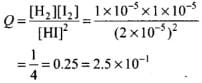Value of Kc = 1 x 10-4
Since Q > Kc, the reaction will proceed in backward direction.

Q.29. On the basis of the equation pH = – log [H+], the pH of 10–8 mol dm–3 solution of HCl should be 8. However, it is observed to be less than 7.0. Explain the reason.
Ans.
Concentration 10-8 mol dm-3 indicates that the solution is very dilute. So, we cannot neglect the contribution of H3O+ ions produced from H2O in the solution. Total [H3O+] = 10-8 + 10-7 M. From this we get the value of pH close to 7 but less than 7 because the solution is acidic.
From calculation, it is found that pH of 10-8 mol dm-3 solution of HC1 is equal to 6.96.

Q.30.pH of a solution of a strong acid is 5.0. What will be the pH of the solution obtained after diluting the given solution a 100 times?
Ans
. pH = 5 i.e., [H+] = 10-5 mol L-1
On dilution by 100 times [H+] = 10-7 mol L-1 For a very dilute solution,
Total [H+] = [H30+ ions from acid] + [H2O+ ions from water]
= 10–7 + 10–7
pH = -log[H+] = -log (2 x 10-7) = 7 – log 2
= 7-0.3010 = 6.6990

Q.31. A sparingly soluble salt gets precipitated only when the product of concentration of its ions in the solution (Qsp) becomes greater than its solubility product. If the solubility of BaSO4 in water is 8 × 10–4 mol dm–3. Calculate its solubility in 0.01 mol dm–3 of H2SO4.
Ans.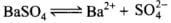At t = 0 1 0 0 At equilibrium in water 1 - S S S At equilibrium in sulphuric acid 1 - S S (S + 0 .0 1 )

Ksp for BaS04 in water = [Ba2+] [SO42-]
= S x S = S2
Ksp = (8 x 10-4)2 = 64 x 10-8
In presence of H2S04, ksp = (S) (S + 0.0 1)
Ksp being constant
(S)(S + 0.01) = 64 x 10-8
S2 + 0.01 S = 64 x 10-8
S2 + 0.01 S - 64 x 10-8 = 0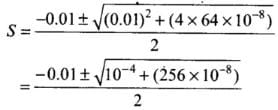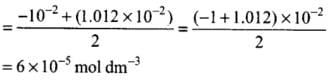Q.32.pH of 0.08 mol dm–3 HOCl solution is 2.85. Calculate its ionisation constant.
Ans. pH of HOC1 = 2.85
-pH = log [H+] or -2.85 = log [H+]
=> [H+] = 1.413 x 10-3

Q.33. Calculate the pH of a solution formed by mixing equal volumes of two solutions A and B of a strong acid having pH = 6 and pH = 4 respectively.
Ans.
pH of solution A = 6
[H+] = 10-6mol L 1
pH of solution B = 4
[H+] = 10-4 molL-1
On mixing one litre of each solution Total volume = 1 L + 1 L = 2 L
Total amount of H+ in 2 L solution formed by mixing solutions A and B = 10-6  + 10-4  mol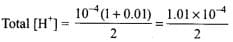= 5 x 10-5 mol L-1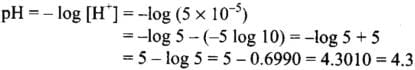Thus, the pH of resulting solution is 4.3.

Q.34. The solubility product of Al (OH)3 is 2.7 × 10–11. Calculate its solubility in gL–1 and also find out pH of this solution. (Atomic mass of Al = 27 u).
Ans.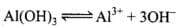Conc, at t = 0 1 0 0 Conc, at equilibrium 1 - S S 3S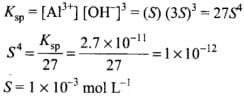(i) Solubility of Al(OH)3
Molar mass of Al(OH)3 = 78 g
∴ Solubility of Al(OH)3 in gL-1
= 1 x 10-3 x 78
= 78 x 10-3
= 7.8 x 10-2 g L-1
(ii) pH o f the solution
S = 1 x 10-3 mol L-1
[OH-1] = 3S = 3 x 1 x 10-3 = 3 x 10-3
pOH = -log [OH-] = - log (3 x 10-3)
pOH = 3 - log 3 = 3 - 0.4771 = 2.552
pH = 14 - pOH = 14 - 2.522 = 11.4771

Q.35. Calculate the volume of water required to dissolve 0.1 g lead (II) chloride to get a saturated solution. (Ksp of PbCl2 = 3.2 × 10–8 , atomic mass of Pb = 207 u).
Ans.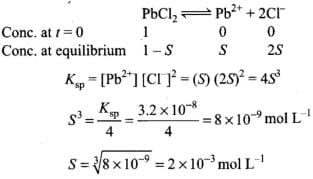Solubility of PbCl2 = 2 x 10-3 x 278 (molar mass of PbCl2) =556 x 10-3gL-1 = 0.556 g L-1
To get saturated solution. 0.556 g PbCl2 is dissolved in 1 L water.
0.1 g o f PbCl2 is dissolved in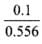= 0.1798 L water
To make a saturated solution, 0.1 g PbCl2 is dissolved in 0.1798 ≌ 0.2 L water

Q.36.A reaction between ammonia and boron trifluoride is given below:
: NH3  +  BF3 → H3N : BF3

Identify the acid and base in this reaction. Which theory explains it? What is the hybridisation of B and N in the reactants?
Ans.
Although BF3 does not have a proton but acts as Lewis acid as it is an electron deficient compound. It reacts with NH3 by accepting the lone pair of electrons from NH3 and completes its octet. The reaction can be represented by
BF3 + :NH3→BF3 ← :NH3
Lewis electro
nic theory of acids and bases can explain it. Boron in BF3 is sp2 hybridised, whereas N in NHis sp3 hybridised.

Q.37.Following data is given for the reaction: CaCO3 (s) → CaO (s) + CO2 (g)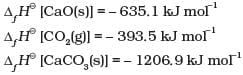Predict the effect of temperature on the equilibrium constant of the above reaction.

Ans.rH° = ∆fH°  [CaO] + ∆fH° [C02] – ∆fH° [CaC03]
= [-635.1] + [-393.5] – [-1206.9] = 178.3 kJ mol-1 Thus, the reaction is endothermic. Hence, according to Le Chatelier’s principle, on increasing the temperature, the equilibrium will proceed in the forward direction.

MATCHING TYPE QUESTIONS

Q.38.Match the following equilibria with the corresponding condition.

 (i) Liquid ⇌ Vapour (a) Saturated solution (ii) Solid ⇌ Liquid (b) Boiling point (iii) Solid ⇌ Vapour (c) Sublimation point (iv) Solute (s) ⇌ Solute (solution) (d) Melting point (e) Unsaturated solution

Ans. (i) →(b), (ii) → (d), (iii) → (c), (iv) → (a)
(i) Liquid ⇌Vapour equilibrium exists at the boiling point.
(ii) Solid ⇌Liquid equilibrium exists at the melting point.
(iii) Solid ⇌ Vapour equilibrium exists at the sublimation point.
(iv) Solute(s) ⇌Solute (solution) equilibrium exists in saturated solution.

Q.39. For the reaction :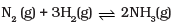Equilibrium constant Kc =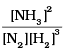Some reactions are written below in Column I and their equilibrium constants in terms of Kc are written in Column II. Match the following reactions with the corresponding equilibrium constant

 Column I (Reaction) Column II (Equilibrium constant) (i)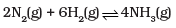(a) 2Kc (ii)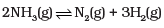(b)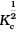(iii)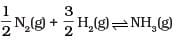(c) 1/Kc (d) Kc2

Ans. (i) →(d), (ii) → (c), (iii) → (b)
Solution.
For N2(g) + 3H2(g) ⇌ 2NH3(g)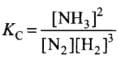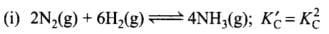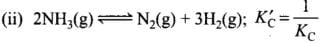Q.40.Match standard free energy of the reaction with the corresponding equilibrium constant.

 (i) ∆ Gθ > 0 (a) K > 1 (ii)  ∆ Gθ < 0 (b) K = 1 (ii)  ∆ Gθ = 0 (c) K = 0 (d) K < 1

Ans.(i) → (d), (ii) → (a), (iii) → (b)
As we know that, ΔG° = - RT In K
(i) If ΔG° > 0, i.e., ΔG° is positive, then In K is negative i.e., K < 1
(ii) If ΔG° < 0, i.e., ΔG° is negative then In K is positive i.e., K > I
(iii) If ΔG° = 0, In K = 0, i.e., K = 1

Q.41.Match the following species with the corresponding conjugate acid

 Species Conjugate acid (i) NH3 (a) CO32– (ii) HCO3– (b) NH4+ (iii) H2O (c) H3O+ (iv) HSO4– (d) H2SO4 (e) H2CO3

Ans.(i) → (b), (ii) → (e), (iii) → (c); (iv) → (d)

Q.42.Match the following graphical variation with their description

 A B (i)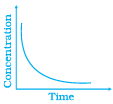(a) Variation in product concentration with time (ii)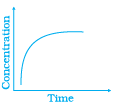(b) Reaction at equilibrium (iii)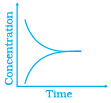(c) Variation in reactant concentration with time

Ans. (i) → (c), (ii) → (a), (iii) → (b)

Q.43.Match Column (I) with Column (II).

 Column I Column II (i) Equilibrium (a) ∆G > 0, K < 1 (ii) Spontaneous reaction (b) ∆G = 0 (iii) Non spontaneous reaction (c) ∆Gθ= 0 (d) ∆G < 0, K > 1

Ans. (i) → (c), (ii) → (d), (iii) → (a)

ASSERTION AND REASON TYPE QUESTIONS

In the following questions a statement of Assertion (A) followed by a statement of Reason (R) is given. Choose the correct option out of the choices given below each question.
Q.44. Assertion (A) : Increasing order of acidity of hydrogen halides is

HF < HCl < HBr < HI
Reason (R) : While comparing acids formed by the elements belonging to the same group of periodic table, H–A bond strength is a more important factor in determining acidity of an acid than the polar nature of the bond.
(i) Both A and R are true and R is the correct explanation of A.
(ii) Both A and R are true but R is not the correct explanation of A.
(iii) A is true but R is false.
(iv) Both A and R are false.
Ans. (i) Both A and R are true and R is the correct explanation of A
Solution.
H-A bond strength is a more important factor in determining acidity than its polar nature. As the size of A increases down the group. H-A bond strength decreases.

Q.45. Assertion (A): A solution containing a mixture of acetic acid and sodium acetate maintains a constant value of pH on addition of small amounts of acid or alkali.
Reason (R):
A solution containing a mixture of acetic acid and sodium acetate acts as a buffer solution around pH 4.75.
(i) Both A and R are true and R is correct explanation of A.
(ii) Both A and R are true but R is not the correct explanation of A.
(iii) A is true but R is false.
(iv) Both A and R are false.
Ans.
(ii) Both A and R are true but R is not the correct explanation of A.
Solution.
The solutions which resist change in pH on dilution or with the addition of small amounts of acid or alkali are called buffer solutions.

Q.46.Assertion (A): The ionisation of hydrogen sulphide in water is low in the presence of hydrochloric acid.
Reason (R) :
Hydrogen sulphide is a weak acid.
(i) Both A and R are true and R is correct explanation of A.
(ii) Both A and R are true but R is not correct explanation of A.

(iii) A is true but R is false
(iv) Both A and R are false
Ans.
(ii) Both A and R are true but R is not correct explanation of A.
Solution.
In presence of H+ ion. equilibrium shifts in backward direction.

Q.47.Assertion (A): For any chemical reaction at a particular temperature, the equilibrium constant is fixed and is a characteristic property.
Reason (R):
Equilibrium constant is independent of temperature.
(i) Both A and R are true and R is correct explanation of A.
(ii) Both A and R are true but R is not correct explanation of A.
(iii) A is true but R is false.
(iv) Both A and R are false.
Ans.
(iii) A is true but R is false.
Solution.

• The equilibrium constant for an exothermic reaction (negative ΔH) decreases as the temperature increases.
• The equilibrium constant for an endothermic reaction (positive ΔH) increases as the temperature increases.

Q.48.Assertion (A): Aqueous solution of ammonium carbonate is basic.
Reason (R):
Acidic/basic nature of a salt solution of a salt of weak acid and weak base depends on Ka and Kb value of the acid and the base forming it.
(i) Both A and R are true and R is correct explanation of A.
(ii) Both A and R are true but R is not correct explanation of A.
(iii) A is true but R is false.
(iv) Both A and R are false.
Ans.
(i) Both A and R are true and R is correct explanation of A.
Solution.
Ka and Kb values are responsible for acidic and basic characters of substances.

Q.49.Assertion (A): An aqueous solution of ammonium acetate can act as a buffer.
Reason (R) :
Acetic acid is a weak acid and NH4OH is a weak base.
(i) Both A and R are true and R is correct explanation of A.
(ii) Both A and R are true but R is not correct explanation of A.
(iii) A is false but R is true.
(iv) Both A and R are false.
Ans.
(iii) A is false but R is true.
Solution.
Salt of weak acid and weak base can form buffer solution.

Q.50. Assertion (A): In the dissociation of PCl5 at constant pressure and temperature addition of helium at equilibrium increases the dissociation of PCl5 .
Reason (R) :
Helium removes Cl2 from the field of action.
(i) Both A and R are true and R is correct explanation of A.
(ii) Both A and R are true but R is not correct explanation of A.
(iii) A is true but R is false.
(iv) Both A and R are false.
Ans. (iii) A is true but R is false.

Solution.
If the volume is kept constant and an inert gas such as argon is added which does not take part in the reaction, the equilibrium remains undisturbed.

Q.51. How can you predict the following stages of a reaction by comparing the value of Kc and Qc?
(i) Net reaction proceeds in the forward direction.
(ii) Net reaction proceeds in the backward direction.
(iii) No net reaction occurs.
Ans.
The values of Kc and Qc are self explanatory and less than or greater than one another decides the direction in which reaction will proceed as follows
(i) As Qc < Kc, the reaction proceeds in the forward direction.
(ii) If Qc > Kc, the reaction will proceed in the direction of reactants (reverse reaction).
(iii) If Qc = Kc, no net reaction occurs.

Q.52.On the basis of Le Chatelier principle explain how temperature and pressure can be adjusted to increase the yield of ammonia in the following reaction.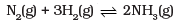∆H = – 92.38 kJ mol-1
What will be the effect of addition of argon to the above reaction mixture at constant volume?

Ans. According to Le Chatelier’s principle, raising the temperature shifts the equilibrium to left and decreases the equilibrium concentration of ammonia since it is an exothermic reaction. In other words, low temperature and high pressure is favorable for high yield of ammonia. There will be no change in equilibria on addition of argon (Ar).

Q.53.A sparingly soluble salt having general formula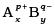and molar solubility S is in equilibrium with its saturated solution. Derive a relationship between the solubility and solubility product for such salt.
Ans.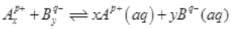S moles of A B dissolve to give x S moles of Ap+ and y S moles of Bq-.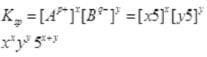Q.54. Write a relation between ∆G and Q and define the meaning of each term and answer the following:
(a) Why a reaction proceeds forward when Q < K and no net reaction occurs when Q = K.

(b) Explain the effect of increase in pressure in terms of reaction quotient Q. for the reaction : CO (g) + 3H2 (g) ⇌ CH4 (g) + H2O (g)
Ans.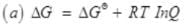ΔGθ = Change in free energy as the reaction proceeds
ΔG = Standard free energy change
Q = Reaction quotient
R = Gas constant
T = Absolute temperature
Since ΔG = -RTInK
∴ ΔG = -RT InK-RT InQ = RT Ik Q/K
If Q < K,ΔG will be negative. Reaction proceeds in the forward.
If Q = K, ΔG = 0, reaction is in equilibrium and no net reaction is there.
(b) On increasing the pressure equilibrium will shift in forward direction it means Q < K.

The document NCERT Exemplar: Equilibrium - Notes | Study Chemistry Class 11 - NEET is a part of the NEET Course Chemistry Class 11.
All you need of NEET at this link: NEET

## Chemistry Class 11

204 videos|331 docs|229 tests

## Chemistry Class 11

204 videos|331 docs|229 tests

### How to Prepare for NEET

Read our guide to prepare for NEET which is created by Toppers & the best Teachers

Track your progress, build streaks, highlight & save important lessons and more!

,

,

,

,

,

,

,

,

,

,

,

,

,

,

,

,

,

,

,

,

,

;